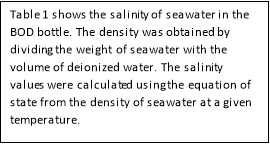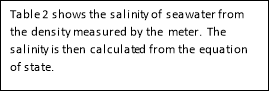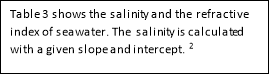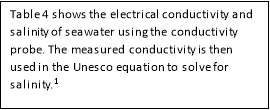Disclaimer: This is an example of a student written essay.

Any scientific information contained within this essay should not be treated as fact, this content is to be used for educational purposes only and may contain factual inaccuracies or be out of date.

# Density and Salinity of Seawater – Comparison of Methods

 ✅ Paper Type: Free Essay ✅ Subject: Chemistry ✅ Wordcount: 2328 words ✅ Published: 24th Jan 2018

Sergian Murtanu, Lab partner: Sammy Chaaban

Abstract: The goal of this experiment was to compare the salinity of seawater from different methods. The average salinity in seawater is around 35 parts per thousand. 3 The BOD bottles were used to find the density of seawater, the equation to state was then applied, using density to determine the salinity. The salinity of seawater from the BOD method was 33.37 ppt ± 0.08737 with a relative standard deviation of 0.2618 percent. Using the same equation of state, the salinity was determined from the readings recorded by the density meter. The density meter produced salinity values of 34.75 ppt ± 1.403, with an RSD% of 4.038. The conductivity probe measured the ability of the solution to pass current. The Unesco equation is used to convert the measured conductivity of seawater to salinity, which was calculated to be 42.71 ppt ± 0.04359 with a RSD% of 0.1021. The refractometer measured the extent of which is bent, giving the refractive index. The average salinity through refractive index was 36.14 ppm ± 2.56, with an RSD% of 0.1884.

Introduction:

The purpose of this experiment was to determine the salinity of seawater through two different methods: BOD bottles (volume) and density meter. Salinity, temperature, and density are related to each other through the equation of state. Physical properties of seawater are related through ‘state functions’. Salinity is defined as a measure of the total dissolved salts in a solution. As density increases, the amount of salt in seawater is expected to increase as well. Thus, density and salinity have a positive relationship with each other. As temperature increases, the area between each water molecule increases, which lowers the density.5 Density has an inverse relationship with temperature. An increase in the salts dissolved in seawater also causes the physical properties of: refractive index, electrical conductivity, transmission of sound, and surface tension to increase. On the contrary, increased salinity in seawater will cause the physical property of: freezing point, compressibility, solubility, and specific heat to decrease instead. Using the methods of electrical conductivity, BOD bottles, density meter, and refractometer, the salinity of seawater will be calculated and compared for the most accurate results. 3

Experimental:

Apparatus:

• BOD Bottles
• Conductivity probe
• Density meter
• Refractometer
• Analytical balances

Procedure:

The density of seawater through the BOD method was discovered by finding the volume of both DI water and seawater. The BOD bottle is weighed dry, and filled with DI water. The temperature of each BOD bottle with DI is taken, and gives a corresponding density value through a chart. Using the equation: Volume=Mass/Density, the volume of DI water can be calculated. This process is repeated with seawater, with density of seawater being the weight of seawater divided by the mean volume of DI water.

If you need assistance with writing your essay, our professional essay writing service is here to help!

The density meter method uses a magnet to measure the period oscillation within the U-shaped tube. Seawater is put in the U-shaped tube. This gives the period of oscillation, T, which is then used in the equation: ρ=AT2 + B , to give the density of seawater(A, B are calibration coefficients). The equation of state given in the spreadsheet, Millero Density Spreadsheet is then used to calculate the salinity at the given density and temperature. 4

The conductivity probe is used to compare the trend between salinity and density. A conductivity probe is first calibrated, then put in a beaker of DI water, standard solution, and seawater. The reading is measured, with the probe cleaned between every new trial. Conductivity is the ability for a solution to pass current, so it is expected that salinity and density have a positive relationship. Using the formula given in the Unesco Equation Spreadsheet, the conductivity ratio is used to calculate the salinity. 1

The refractometer is a tool that measures the extent in which light is bent. Drop a sample of seawater to the measuring prism and use the hand wheel to adjust the sight. The illuminating prism should be put at the halfway point of the sample, this then gives the refractive index. Using given slope and intercept “Salinity vs. Refractive Index” and “Salinity vs. Specific Gravity” Curves, the salinity and specific gravity is then calculated. 2

Results:Table 1 – Seawater salinity in BOD Bottle

 Trial Temperature (C) Salinity 1 21.5 33.35 2 21.6 33.47 3 21.7 33.30 Mean Salinity 33.37 Standard Deviation 0.08737 Relative Standard Deviation % 0.2618

Table 2 – Salinity of seawater by density meter – Data obtained by Jessica Oregon

 Trial Temperature (C) Salinity 1 2033.53 2 20 35.95 3 20 33.53 4 20 35.97 Mean Salinity 34.75 Standard Deviation 1.403 Relative Standard Deviation % 4.038

Table 3 – Salinity of seawater by refractive index

 Trial SeawaterSalinity 1 1.3400 38.37 2 1.3391 33.34 3 1.3397 36.69 Mean Salinity 36.14 Standard Deviation 2.560 Relative Standard Deviation % 7.083

Table 4 – Salinity of seawater by conductivity probe (22.6 C)

 Trial Seawater (mS/cm) Standard (mS/cm) Salinity 1 49.6 49.4 42.76 2 49.6 49.5 42.68 3 49.7 49.6 42.68 4 49.6 49.4 42.76 5 49.7 49.6 42.68 Mean 49.6 49.5 42.71 Standard Deviation 0.0548 0.100 0.0436 Relative Standard Deviation % 0.110 0.202 0.102Discussion:

The results seem to imply that the salinity in seawater varies depending on the method, as some methods have a lesser chance of error than others. Using the average seawater salinity of 35 ppt as a comparison, most of the results seem to fall between the ranges of 33 to 37. However, the most notable difference is the salinity of seawater by conductivity probe. The conductivity probe method produced a mean salinity of 42.71 ppt ± 0.0436, with a relative standard deviation of 0.102 percent. The results for the conductivity probe were quite far in comparison to the other method. However, the conductivity method had an RSD% of 0.1021. This indicated that the results while somewhat inaccurate, were very precise. This inaccuracy may have been caused due to systematic errors in our instrument. The conductivity probe might have been calibrated incorrectly, or in the wrong solution. If the calibration was just slightly off, it is possible that this difference might have contributed to the inaccurate salinity results. The solutions measured on the probe might have gotten contaminated without prior knowledge, or it might have simply been a human error in calculations.

Our academic experts are ready and waiting to assist with any writing project you may have. From simple essay plans, through to full dissertations, you can guarantee we have a service perfectly matched to your needs.

The result with the highest relative standard deviation was the salinity of seawater by refractive index with 7.083 %. This indicates that there was a lot of variation in salinity for each trial. The salinity ranges from 33.34 to 38.37 ppt. The mean salinity of seawater by refractive index was 36.14 ppt ± 2.560, which is close to the average seawater salinity of 35. These slightly imprecise results may be due to random and systematic errors. The use of the eyepiece when trying to go to the halfway point might change due to different perception between people. Likewise, reading the refractometer varies between each individual and might change with angle due to parallax. This can really change the data measured and can result in imprecise data. There can also be human errors done during the conversion between refractive index and salinity.

The BOD bottle method has a salinity of 33.37 ppt ± 0.08737 with an RSD% of 0.2618. The very low relative standard deviation indicates that there isn’t much spread within the data. When compared to the average seawater salinity of 35 ppt, it falls a little bit short. This was because there are a lot of possible errors for this method due to the long process of balancing and rebalancing. Possible systematic errors might have occurred on the analytical balances, such as calibration. Random errors, such as the fluctuation of weight in our seawater sample might also have impacted the accuracy. This method was also very time consuming, so it was not the most efficient way of determining the salinity. But, based on the results, it was the most dependable with good accuracy and great precision.

The most accurate method when compared to the average value of seawater salinity is the density meter. The density meter has a salinity of 34.75 ± 1.403, with an RSD% of 4.038. The RSD% of 4.038 indicated that there was some variation in the data. However, when compared to the average seawater salinity, this was the closest by far. This method was the most efficient overall because it allowed the opportunity to obtain the density, and salinity value the quickest. However, it’s not perfect because the instrument can calibrated or used incorrectly.

While the most precise method goes to the BOD method, the most accurate would go to the density meter. The density meter is also quick and efficient, which make it an excellent tool to calculate the value for salinity. In comparison, the BOD method took far too long and was not nearly as efficient as the density meter method. If there was one thing that should be changed in a repeat of this experiment, it would be giving an extended time for the density meter method. There was not enough time in the assigned lab period, so data from a peer was borrowed and used.

Acknowledgements:

I would like to thank Sam and George for helping us with calculations. I would also like to thank fellow peer, Jessica Oregon for the data set on seawater density by meter.

References:

1. Anderson, George, and Michael Tauber.Unesco Density Spreadsheet. University of California, San Diego: Ted.ucsd.edu, n.d. Xlsx.
1. Tauber, Michael, and George Anderson.“Salinity vs Refractive Index” and “Salinity vs Specific Gravity” Curves. University of California, San Diego: Ted.ucsd.edu, n.d. Xlsx.
1. Tauber, Michael, and Robert Pomeroy. “3.5 Density and Salinity lecture.”Ted.ucsd.edu. N.p., n.d. Web. 02 Feb. 2015.
1. Tauber, Michael, and Robert Pomeroy.Milero Density Spreadsheet. University of California, San Diego: Ted.ucsd.edu, n.d. Xlsx.
1. “The Ocean and Temperature.”The Ocean and Temperature. N.p., n.d. Web. 02 Feb. 2015.

View all

## DMCA / Removal Request

If you are the original writer of this essay and no longer wish to have your work published on UKEssays.com then please:

Related Services

Prices from

S\$203

Approximate costs for:

• 1000 words
• 7 day deliveryHumanity University

Dedicated to your worth and value as a human being!

Related Lectures